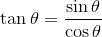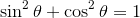Search IntMath
Close

# Trig ratios: updated interactive

By Murray Bourne, 01 Jul 2013

Math teachers tend to present the trigonometric identities to students and expect instant understanding and acceptance.

It would be better in many cases to let the students discover for themselves how such identities work. We don't always have the luxury of time for exploration in most math classrooms, but it is something that we should try to do.

I just updated an interactive graph that allows students to explore 2 key trig identities, for any angle θ:andHere it is: Trigonometric Ratios - Interactive Graph

Let me know if it is useful for you, or your students. Your suggestions are always welcome, too.

### Comment Preview

HTML: You can use simple tags like <b>, <a href="...">, etc.

To enter math, you can can either:

1. Use simple calculator-like input in the following format (surround your math in backticks, or qq on tablet or phone):
a^2 = sqrt(b^2 + c^2)
(See more on ASCIIMath syntax); or
2. Use simple LaTeX in the following format. Surround your math with $$ and $$.
$$\int g dx = \sqrt{\frac{a}{b}}$$
(This is standard simple LaTeX.)

NOTE: You can mix both types of math entry in your comment.